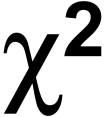Martin’s recent article on inking his new GameScience dice naturally led to a spirited discussion about GameScience’s claims that their dice are the best dice available in terms of randomness, which quite naturally leads one to ask: “Is my favorite die fair? How can I tell?” One possibility is to perform a chi*-square goodness of fit test. This doesn’t include any difficult math, though it can be tedious without a spreadsheet program.

The purpose of a goodness of fit test (often called simply a chi-square test, though this is a misnomer since there are many forms of chi-square tests, not all of which are goodness of fit tests) is to test the claim that a process produces results in some specified frequency.Â  In this case, we’re testing to see if every face on our die comes up an equal number of times.

To do this, the usual procedure is to set up a table with a row for each possible outcome and a column for each of the following:Â  Expected number of results in an outcome (denoted “E”), Observed number of results in an outcome (denoted “O”), O-E, (O-E)^2, and [(O-E)^2]/E.Â  Here’s an example table setup for a d20: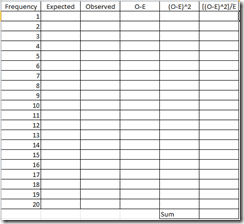Once your table is set up, Decide how many times you’re going to roll your die, and fill in the expected frequencies. The minimum number of times you need to roll the die is the number that makes the expected number of outcomes for each category 5 or more.Â  Since dice are designed to have an even chance to roll each number, the minimum number for testing dice is 5 times the number of sides.Â  A larger sample will improve the accuracy of your results, but this has a diminishing rate of returns, so there’s no reason to go overboard and roll your die thousands of times.Â  Here’s an example table with expected and observed frequencies for our d20: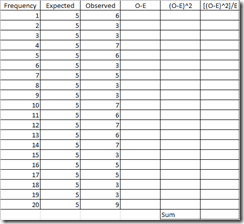Note that since we’re testing a d20 and we want an expected value of at least 5 in every frequency, we’ve rolled our die 100 times (20×5=100).Â  If we wanted, we could roll 200 times and use and expected value of 10, but that’s not really necessary.

Now that you have the observed and expected frequencies, it’s time to fill in the remaining columns in the table.Â  Each one is based on earlier ones, so to fill in O-E for the first row, you simply take the observed frequency from the first row and subtract the expected frequency from the first row.Â  to find (O-E)^2, you take the O-E column from that row and square it, and to find the [(O-E)^2]/E for a row you take that row’s (O-E)^2 column and divide by that Row’s E.Â  Finally you sum up all the values from the [(O-E)^2]/E column.Â  Here’s an example of what that will look like: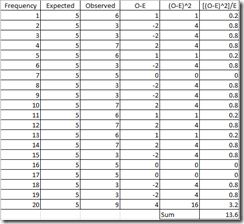The summed total of the [(O-E)^2]/E column is called your chi-square test statistic.Â  To test the claim that your die is fair, you have to compare it to a chi-square critical value from a chi-square table.Â  To find your critical value, you need to know two things: Your Alpha and your degrees of freedom.Â  Alpha is statistician talk for “What proportion of the time am I willing to be wrong if my claim is actually true?” and industry standards are .1, .05 and .01. Since being wrong testing your die isn’t really a big deal, we’ll choose .1 as our default Alpha, but if you want to have less risk of being incorrect, use a smaller one.Â  Degrees of Freedom is calculated differently for different tests, but for this one it’s #of categories in your goodness of fit table – 1.Â  So for our d20 above, we have 19 degrees of freedom.

On the chi-square table, columns are different alpha’s (called P on the chart I linked) and rows are different Degrees of Freedom.Â  Find the value where yours intersect. This value is your critical value.Â  For Alpha=.1 and df=19 our critical value is 27.204.

Now we compare our test statistic to our critical value. We want to know which is larger.Â  If our test statistic is larger, this is evidence that our die is NOT fair, so we reject the claim that our die is fair.Â  If our critical value is larger, we do not have evidence that our die is not fair, so we fail to reject the claim that our die is fair.Â  In our example, our test statistic is 13.6. Our critical value is larger than this, so we fail to reject the claim that this is a fair die.

Here’s another set of data with a test statistic of 31.6.Â  In this case, the test statistic is larger than the critical value, so we will reject the claim that the die is fair: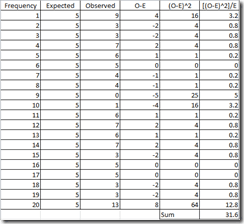And that’s all there is to the chi-square goodness of fit test!Â  While the vast majority of dice are fair, there’s always the chance that your “lucky die” really is “lucky” and this is the tool to find out.

*To the best of my knowledge, chi is pronounced “kai” (hard k, long I as in Cobra-Kai dojo), not “key” (as in housekey), nor “chee” (as in mun-chee-chee), nor “chai” (as in chai-tea latte). However, if your greek is better than mine (ie: you know anything about greek at all) feel free to correct me in the comments.